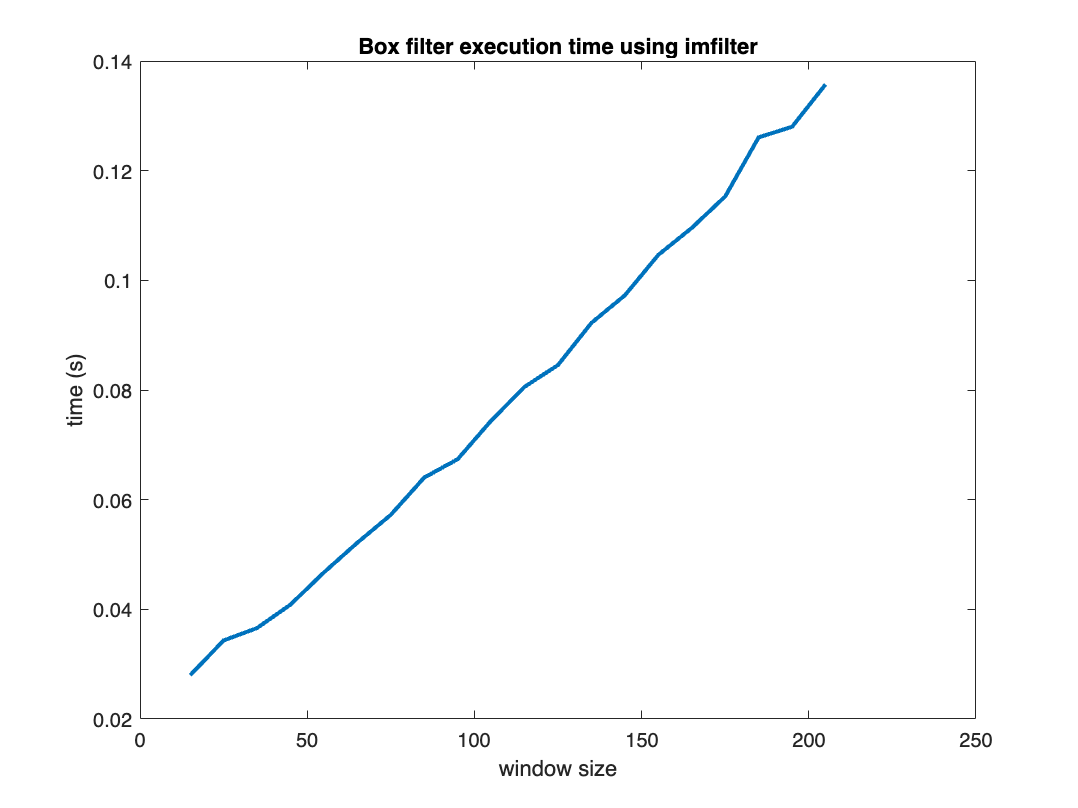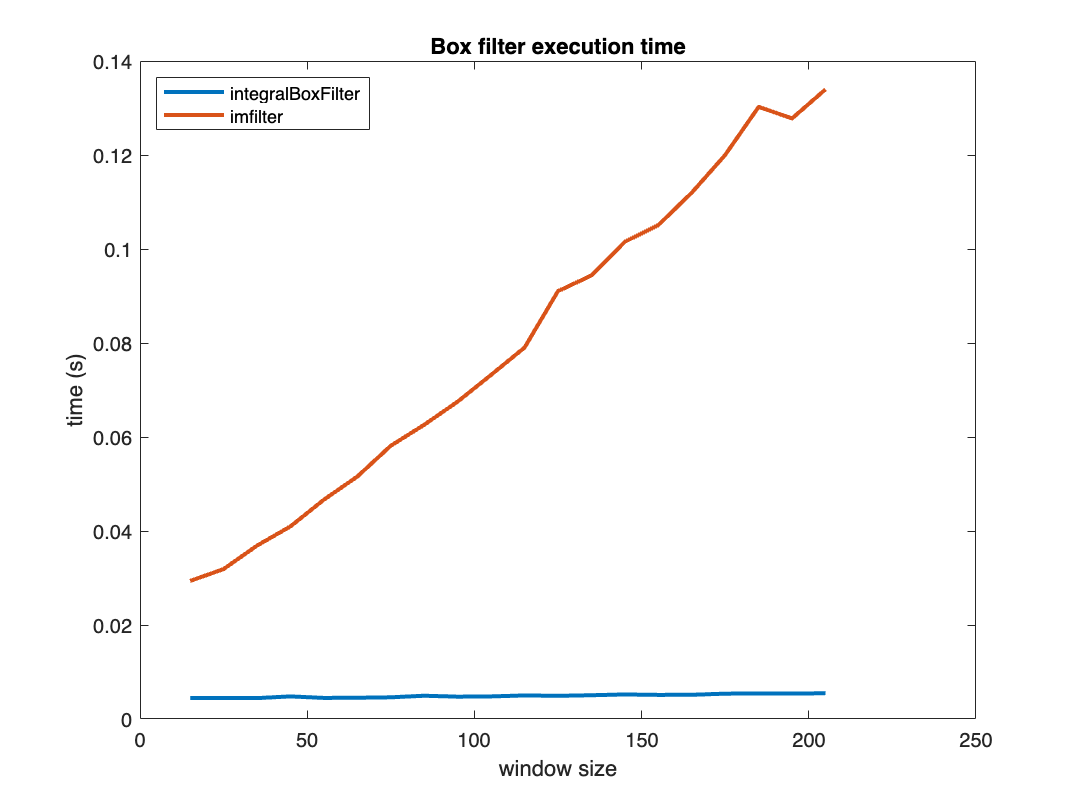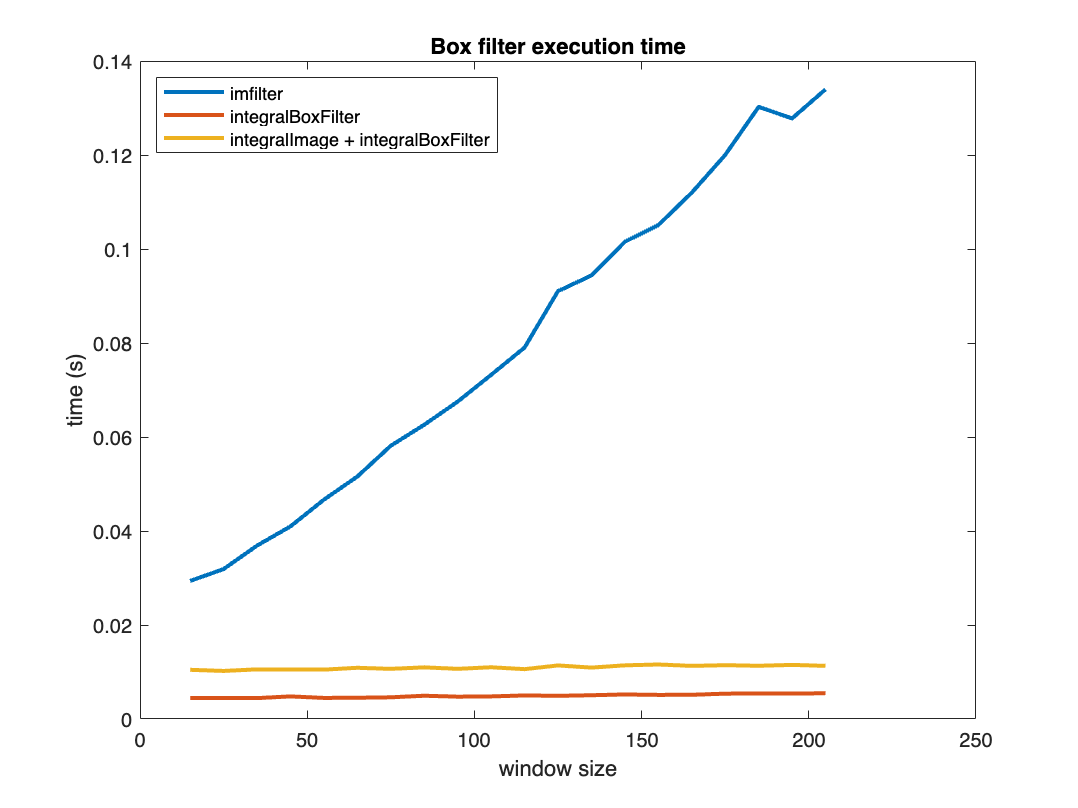# Fast Local Sums, Integral Images, and Integral Box Filtering

In May 2006, I wrote about a technique for computing fast local sums of an image. Today, I want to update that post with additional information about integral image and integral box filtering features in the Image Processing Toolbox.
First, let's review the concept of local sums.
A = magic(7)
A = 7×7
30 39 48 1 10 19 28 38 47 7 9 18 27 29 46 6 8 17 26 35 37 5 14 16 25 34 36 45 13 15 24 33 42 44 4 21 23 32 41 43 3 12 22 31 40 49 2 11 20
The local sum of the $3 \times 3$ neighborhood around (2,3) element of A can be computed this way:
submatrix = A(1:3, 2:4)
submatrix = 3×3
39 48 1 47 7 9 6 8 17
local_sum_2_3 = sum(submatrix,"all")
local_sum_2_3 = 182
In image processing applications, we are often interested in the local sum divided by the number of elements in the neighborhood, which is 9 in this case:
local_sum_2_3/9
ans = 20.2222
Computing all the local sums for the matrix, divided by the neighborhood size, is the same as performing 2-D filtering with a local mean filter, like this:
B = imfilter(A,ones(3,3)/9);
B(2,3)
ans = 20.2222
This is often called a box filter. Computing an $N \times N$ box filter for an entire $P \times Q$ image would seem to require approximately $PQN^2$ operations. In other words, for a fixed image size, we might expect the box filter computational time to increase in proportion to $N^2$, where N is the local sum window size.
Let's see how long imfilter takes with respect to a changing local sum neighborhood size.
I = rand(3000,4000);
nn = 15:10:205;
imfilter_times = zeros(size(nn));
for k = 1:length(nn)
n = nn(k);
f = @() imfilter(I,ones(n,n)/n^2);
imfilter_times(k) = timeit(f);
end
plot(nn,imfilter_times)
title("Box filter execution time using imfilter")
xlabel("window size")
ylabel("time (s)")The function imfilter is doing better than expected, with an execution time that increases only linearly with the window size. (That's because imfilter is taking advantage of filter separability, which I have written about previously.)
But we can compute local sums even faster, by using something called an integral image, also known as a summed-area table. Let's go back to the magic square example and use the function integralImage.
Ai = integralImage(A)
Ai = 8×8
0 0 0 0 0 0 0 0 0 30 69 117 118 128 147 175 0 68 154 209 219 247 293 350 0 114 206 269 296 350 431 525 0 119 225 304 356 444 561 700 0 132 253 356 441 571 732 875 0 153 297 432 558 731 895 1050 0 175 350 525 700 875 1050 1225
Each element of Ai is the cumulative sum of all the elements in A that are above and to the left. Ai(4,3), which is 206, is the sum of all the elements of A(1:3,1:2).
A(1:3,1:2)
ans = 3×2
30 39 38 47 46 6
sum(ans,"all")
ans = 206
Ai(4,3)
ans = 206
Expressed as a general formula, the integral image is $A_i(m,n) = \sum_{p=1}^{m-1} \sum_{q=1}^{n-1} A(p,q)$.
The really interesting thing about having the integral image, Ai, is that it lets you compute the sum of any rectangular submatrix of A by an addition and subtraction of just four numbers. Specifically, the sum of all the elements in A(m1:m2,n1:n2) is Ai(m2+1,n2+1) - Ai(m1,n2+1) - Ai(m2+1,n1) + Ai(m1,n1). Consider computing the sum of all elements in A(3:5,2:6).
m1 = 3;
m2 = 5;
n1 = 2;
n2 = 6;
A(m1:m2,n1:n2)
ans = 3×5
6 8 17 26 35 14 16 25 34 36 15 24 33 42 44
sum(ans,"all")
ans = 375
Ai(m2+1,n2+1) - Ai(m1,n2+1) - Ai(m2+1,n1) + Ai(m1,n1)
ans = 375
If we have the integral image, then, we can compute every element of the box filter output by adding just four terms together. In other words, the box filter computation can be independent of the box filter window size!
The Image Processing Toolbox function integralBoxFilter does this for you. You give it the integral image, and it returns the result of the box filter applied to the original image.
Let's see how long that takes as we vary the box filter window size.
integral_box_filter_times = zeros(1,length(nn));
for k = 1:length(nn)
n = nn(k);
h = @() integralBoxFilter(Ai,n);
integral_box_filter_times(k) = timeit(h);
end
plot(nn,integral_box_filter_times, ...
nn,imfilter_times)
legend(["integralBoxFilter" "imfilter"], ...
Location = "northwest")
title("Box filter execution time")
xlabel("window size")
ylabel("time (s)")That looks great! As expected, integralBoxFilter is fast, with execution time that is independent of the window size. To be completely fair, though, we should include the time required to compute the integral image.
integral_box_filter_total_times = zeros(1,length(nn));
for k = 1:length(nn)
n = nn(k);
r = @() integralBoxFilter(integralImage(I),n);
integral_box_filter_total_times(k) = timeit(r);
end
plot(nn,imfilter_times,...
nn,integral_box_filter_times,...
nn,integral_box_filter_total_times)
title("Box filter execution time")
xlabel("window size")
ylabel("time (s)")
legend(["imfilter" "integralBoxFilter" "integralImage + integralBoxFilter"],...
Location = "northwest")From the plot, we can see that using the integral image for box filtering is the way to go, even when you take into consideration the time needed to compute the integral image.
|